Dynamic optimization model for allocating medical resources in epidemic controlling

Dynamic optimization model for allocating medical resources in epidemic controlling

Ming Liu1, Jing Liang2

1Nanjing University of Science and Technology, 2Nanjing College of Chemical Technology (China)

Accepted February 2013

Liu, M., & Liang, J. (2013). Dynamic optimization model for allocating medical resources in epidemic controlling. Journal of Industrial Engineering and Management, 6(1), 73-88. http://dx.doi.org/10.3926/jiem.663

---------------------

Abstract:

Purpose: The model proposed in this paper addresses a dynamic optimization model for allocating medical resources in epidemic controlling.

Design/methodology/approach: In this work, a three-level and dynamic linear programming model for allocating medical resources based on epidemic diffusion model is proposed. The epidemic diffusion model is used to construct the forecasting mechanism for dynamic demand of medical resources. Heuristic algorithm coupled with MTLAB mathematical programming solver is adopted to solve the model. A numerical example is presented for testing the model’s practical applicability.

Findings: The main contribution of the present study is that a discrete time-space network model to study the medical resources allocation problem when an epidemic outbreak is formulated. It takes consideration of the time evolution and dynamic nature of the demand, which is different from most existing researches on medical resources allocation.

Practical implications: In our model, the medicine logistics operation problem has been decomposed into several mutually correlated sub-problems, and then be solved systematically in the same decision scheme. Thus, the result will be much more suitable for real operations.

Originality/value: In our model, the rationale that the medical resources allocated in early periods will take effect in subduing the spread of the epidemic spread and thus impact the demand in later periods has been for the first time incorporated. A win-win emergency rescue effect is achieved by the integrated and dynamic optimization model. The total rescue cost is controlled effectively, and meanwhile, inventory level in each urban health departments is restored and raised gradually.

Keywords: epidemic diffusion, time-varying demand, time-space network, dynamic optimization

---------------------

1. Introduction

As mentioned in (Rachaniotis, Dasaklis & Pappis, 2012), a serious epidemic is a problem that tests the ability of a nation to effectively protect its population, to reduce human loss and to rapidly recover. Sometime such a problem may acquire worldwide dimensions. For example, during the period from November 2002 to August 2003, 8422 people in 29 countries were infected with SARS, 916 of them were dead at last for the effective medical resources appeared late. Other diseases, such as HIV, H1N1 also cause significant numbers of direct infectious disease deaths.

Actually, many recent research efforts have been devoted to understanding the prevention and control of epidemics, such as Wein, Liu and Leighton (2005), Craft, Wein and Alexander (2005) and Kaplan, Craft and Wein (2002). The major purpose of these articles is to compare the performance of the following two strategies, the traced vaccination (TV) strategy and the mass vaccination (MV) strategy, but not address how to optimize the allocation of medical resources.

Another stream of research is on the development of epidemic diffusion models by applying complex network theory to traditional compartment models. For example, Saramäki & Kaski (2005) proposed a susceptible-infected-recovered (SIR) model for the spreading of randomly contagious diseases, such as influenza, based on a dynamic small-world network. Xu, Peng, Wang and Wang (2006) presented a modified susceptible-infected- susceptible (SIS) model based on complex networks, small-world and scale-free, to study the spread of an epidemic by considering the effect of time delay. Based on two-dimensional small-world networks, a susceptible-infected (SI) model with epidemic alert is proposed in (Han, 2007). This model indicates that to broadcast an epidemic alert timely is helpful and necessary in the control of epidemic spreading. Jung, Iwami and Takeuchi (2009) extended the previous studies on the prevention of the pandemic influenza to evaluate the time-dependent optimal prevention policies, and they found that the quarantine policy is very important, and more effective than the elimination policy. After determining the epidemiologic features of an Escherichia coli O157:H7 outbreak in Xuzhou, Jiangsu Province, China, Zhu, Gu, Yu et al. (2009) provided a scientific approach for establishing prevention and control strategies in local areas. These above mentioned works represent some of the research on various differential equation models for epidemic diffusion and control.

However, after an epidemic outbreak, public officials are faced with many critical issues, one of the most important of which being how to ensure the availability and supply of medical resources so that the loss of life may be minimized and the rescue operation efficiency maximized. Sheu (2007) presented a hybrid fuzzy clustering-optimization approach to the operation of medical resources allocation in response to the time-varying demand during the crucial rescue period. Yan and Shih (2009) considered how to minimize the length of time required for emergency roadway repair and relief distribution, as well as the related operating constraints. The weighting method is adopted, and a heuristic algorithm is developed to solve a real emergency relief problem, the Chi-Chi earthquake in Taiwan. To optimize the process of materials distribution in an epidemic diffusion system and to improve the distribution timeliness, Liu and Zhao (2009) modeled the emergency materials distribution problem as a multiple traveling salesman problem with time window. Wang, Wang and Zeng (2009) constructed a multi-objective stochastic programming model with time-varying demand for the emergency logistics network based on the epidemic diffusion rule. A genetic algorithm coupled with Monte Carlo simulation is adopted to solve the optimization model. Recently, Qiang and Nagurney (2011) proposed a humanitarian logistic model for supply/distribution of critical needs in a disruption caused by a nature disaster. They consider a general network structure and disruptions that may have an impact to both network link capacities and product demand. The problem is studied in a bi-criteria system optimization framework for network performance.

In this article, a three-level and dynamic linear programming model for allocating medical resources based on epidemic diffusion model is proposed. The epidemic diffusion model introduced here is to construct the forecasting mechanism for the dynamic demand of medical resources. Heuristic algorithm coupled with MTLAB mathematical programming solver is adopted to solve the model. The remainder of the article is organized as follows: Section 2 is the problem description, including the time-varying demand forecasting model based on the epidemic diffusion rule for the infected areas and the time-varying demand forecasting model for the local health departments. The three-level and dynamic optimization model and the solution procedure are introduced in Section 3. A numerical example is presented in Section 4. Finally, the limitations of the proposed model and future research directions are proposed in Section 5.

2. Problem description

2.1. The research ideas and way to achieve

As work in Liu and Zhao (2011), this article focus on the recovered stage of epidemic rescue. In such a stage, epidemic diffusion tends to be stable. Thus, optimization goal in such stage is to construct an integrated, dynamic and multi-level emergency logistics network, which includes the national strategic storages (NSS), the urban health departments (UHD), the area disease prevention and control centers (ADPC), and the emergency designated hospitals (EDH). Herein, we introduce a time-space network to depict the network structure relationship of these elements, which is shown as Figure 1. In such figure, the vertical axis represents the time duration, and the horizontal axis represents different emergency departments.Figure 1. Time-space network of medical resources allocation

The entire recovered stage of epidemic rescue process is decomposed into several mutually correlated sub-problems (i.e. n decision-making cycles). To each decision-making cycle, there exist two sub-problems. In the upper level, we consider the problem how to replenish medical resources to the UHDs. Besides, we adjust the replenishment arcs among these NSSs by a heuristic algorithm, and construct a mixed-collaborative delivery system. Thus, the total rescue cost of the upper level sub-problem would be minimized. In the lower level, we present the problem how to distribute medical resources to the ADPCs and then allocate medical resources to EDHs. We propose a forecasting model for the time-varying demand in EDHs based on a SEIR epidemic diffusion model. Such two phases are executed iteratively. Besides, at the end of each rescue cycle, effect of medical resources allocated is analyzed and the number of infected people is updated. The research idea of such rescue stage is shown as Figure 2.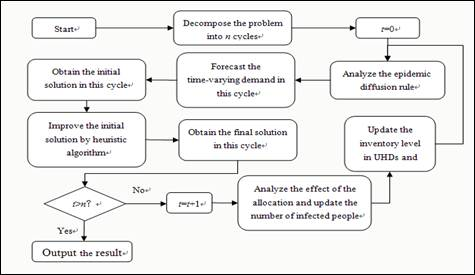Figure 2. Operational procedure of the dynamic medicine logistics network

2.2. Time-varying forecasting method for the dynamic demand

In this article, we divide people into four classes: the susceptible people (S), the exposed people (E), the infected people (I), and the recovered people (R). The following SEIR epidemic diffusion model in Liu and Zhao (2012) is adopted to depict the epidemic diffusion rule.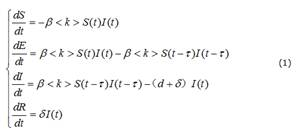Herein, <k> is the average degree distribution of the small-world network; b is the propagation coefficient; d is the recovered rate of the infected people; d is the death rate caused by the disease; stands for the incubation period. Furthermore, <k>,b,d,d,t>0. Traditionally, a simple linear function is used to formulated the demand for medical resources as follows: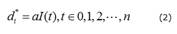Herein, I(t) is the number of infected people in the epidemic area at time t. a is the proportionality coefficient. Note that emergency demand for medical resources is closely related to the number of infected people, and medical resources allocated in the early rescue cycle will affect the demand later, here we propose a time-varying forecasting method for the demand in each EDH as follows: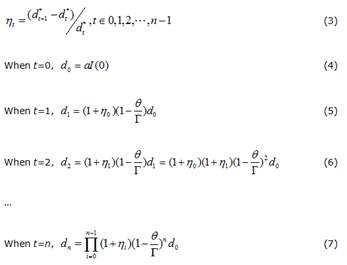Herein,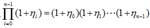. q is the effective rescue rate; G is the treatment cycle for each infected person. To facilitate the calculation process in the following sections, we assume that G to be an integral multiple of rescue cycle. Equation (3) is used to calculate the linear scale factor of the change in demand. Equation (4) is the initial demand in the epidemic area, and I(0) represents the initial number of infected people in the epidemic area. Equations (5)-(7) represent demand for medical resources in rescue cycle 1,2,…,n, respectively.

2.3. Dynamic demand and inventory for the UHD

To facilitate the calculation process in the following sections, we assume that initial inventory of medical resources in each UHD is zero. Besides, we suppose that capacity of each UHD is Vcap · dtv represents demand for medical resources in UHD in rescue cycle t. Pt represents the total output of medical resources in UHD in rescue cycle t. Thus, the forecasting model for dynamic demand in UHD can be formulated as follows: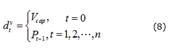Correspondingly, suppose that Vt is inventory of medical resources in UHD in rescue cycle t, we can get the following equation: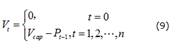3. Optimization model and solution procedure

3.1. Optimization model

The following assumptions are needed to facilitate the model formulation in the following sections:

(1) Once an epidemic outbreak, each EDH can be isolated from other areas to avoid the spread of the disease.

(2) It is reasonable to assume that the government can ensure the adequate supply of the needed medicines either from domestic pharmaceutical companies or imported. Hence, there are enough medical resources during the entire operation process.

(3) Holding cost of medical resources is not considered in this paper for two reasons.

(4) Medical resources in this section are an assembled product, which may includes water, vaccine, antibiotic, etc.

Notations used in the following optimization model are specified as follows.

ncij: Unit replenishment cost of medical resources from NSS i to UHD j.

cejk: Unit distribution cost of medical resources from UHD j to ADPC k.

ehkl: Unit distribution cost of medical resources from ADPC k to EDH l.

nsi: Amount of medical resources supplied by NSS i in each rescue cycle.

Vcap: Capacity of UHD.

dlt: Demand for medical resources in EDH l in rescue cycle t.

djtv: Demand for medical resources in UHD j in rescue cycle t.

Pjt: Total output of medical resources in UHD j in rescue cycle t.

Vjt: Inventory of medical resources in UHD j in rescue cycle t.

xjt: Amount of medical resources that will be transported from NSS i to UHD j in rescue cycle t.

yjkt: Amount of medical resources that will be transported from UHD j to ADPC k in rescue cycle t.

zklt: Amount of medical resources that will be transported from ADPC k to EDH l in rescue cycle t.

TC: Total rescue cost of the three-level medical logistics network.

N: Set of NSSs.

C: Set of UHDs.

H: Set of EDHs.

T: Set of decision-making cycles.

According to the above explanations and assumptions, the three-level and dynamic linear programming model for allocating medical resources based on epidemic diffusion model can be formulated as follows: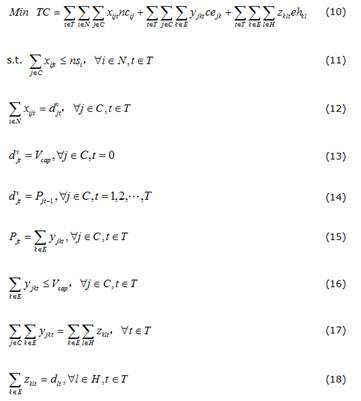Herein, the objective function in Equation (10) is to minimize the total rescue cost of the three-level medical distribution network. Equations (11) and (12) are constraints for flow conservation in the upper level sub-problem. Equations (13)-(15) are the dynamic demand models in the upper level sub-problem. Equations (16)-(18) are constraints for flow conservation in the lower level sub-problem. Equations (19)-(21) are the time-varying demand models in the lower level sub-problem. At last, Equations (21)-(23) ensure all the arc flows in the time-space network within their bounds.

3.2. Solution procedure

As Figure 2 shows, the solution procedure for the proposed optimization model is presented as follows:

Step 1. Decompose the entire recovered stage of epidemic rescue process into n decision-making cycles.

Step 2. Let t=0, and initialize parameters in the SEIR epidemic diffusion model.

Step 3. Analyze the epidemic diffusion rule, and calculate the initial demand for medical resources in each EDH according to Equations (3)-(7).

Step 4. Solve the programming model in rescue cycle t=0 and obtain the initial solution.

Step 5. Improve the initial solution by heuristic algorithm. Detail about the heuristic algorithm, please go to Liu, Li and Chan (2003).

Step 6. Get the final solution for medical resources allocation in this rescue cycle.

Step 7. Let t=t+1, if the termination condition for the rescue cycle has not been satisfied, update the demand in each EDH, and update the inventory level of medical resources in each UDH, go back to Step 3. Else, go to the next step.

Step 8. End the program and output the final result.

4. Numerical example

To test how well the proposed model may be applied in an actual event, we present a numerical example to illustrate its efficiency. Assume there is a smallpox outbreak in a city, which has 3 NSSs, 4 UHDs, 6 ADPCs and 8 EDHs. The values of parameters in SEIR epidemic diffusion model are given in Table 1.

Figure 3 depicts a numerical simulation of the epidemic model at EDH 1 in this effected region. The four curves respectively represent the number of four groups of people (S, E, I, R) over time. As mentioned in , the process of epidemic diffusion is divided into three stages and our research focus on the recovered one. According to the above figure, we assume that such stage range from the 45th day (decision-making cycle t=0) to the 55th day (decision-making cycle t=10). For simplicity, the decision-making cycle is assumed to be one day. A total of 138240 variations are generated in the experiment. During an actual epidemic activity, decision makers can adjust these parameters according to the actual situation.

 EDH1 EDH2 EDH3 EDH4 EDH5 EDH6 EDH7 EDH8 S(0) 5x103 4.5x103 5.5x103 5x103 6x103 4.8x103 5.2x103 4x103 E(0) 30 35 30 40 25 40 50 45 I(0) 5 6 7 8 4 7 9 10 R(0) 0 b 4x10-5 6 d 0.3 d 1x10-3 t 5

Table 1. Values of parameters in SEIR epidemic diffusion model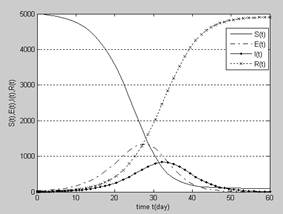Figure 3. Solution of the SEIRS epidemic diffusion model (EDH 1)

Let a=1, q=90% and G=15, the MATLAB mathematic solver coupled with Equations (2)-(7) is adopted to forecast the time-varying demand for each EDH. Taking the EDH 1 as an example, the demand for medical resources in each rescue cycle is shown in Figure 4. One can observe in Figure 4 that time-varying demand is way below traditional demand, suggesting that the allocation of medical resources in the early periods will significantly reduce the demand in the following periods. The second observation is that both curves exhibit similar trends, namely, the demand will decrease after the epidemic is brought under control.

After getting the demand for medical resources in each rescue cycle, in what follows, we are going to discuss how to allocate these medical resources to the epidemic areas, and at the same time, how to replenish medical resources to each UHD, with the objective of minimizing the total rescue cost. Table 2 shows the unit cost from the supply points to the demand points (Here, we suppose that NSS 1 has been preset as the HUB location).Figure 4. Demand in EDH 1 by the two different methods

 cost N1 C1 C2 C3 C4 N1 ---- 2 2 1 3 N2 3 7 2 10 9 N3 4 8 9 2 10 E1 E2 E3 E4 E5 E6 C1 1 3 4 2 5 5 C2 2 1 2 3 5 4 C3 2 3 5 4 1 3 C4 4 4 1 2 3 2 H1 H2 H3 H4 H5 H6 H7 H8 E1 6 2 6 7 4 2 5 9 E2 4 9 5 3 8 5 8 2 E3 5 2 1 9 7 4 3 3 E4 7 6 7 3 9 2 7 1 E5 2 3 9 5 7 2 6 5 E6 5 5 2 2 8 1 4 3

Table 2. Unit cost of medical resources between two different departments

Assume that three NSS can supply 400, 420 and 450 unit of medical resources in each rescue cycle, and suppose that capacity of each UHD is 320. Let us take the allocation result at cycle t=0 as example, we can solve the programming model according to the solution procedure. The initial solution is reported in Table 3.

 Amount C1 C2 C3 C4 Before adjustment N1 100 0 0 320 N2 110 320 0 0 N3 110 0 320 0 N1 C1 C2 C3 C4 After adjustment N1 ---- 320 0 0 320 N2 110 0 320 0 0 N3 110 0 0 320 0

Table 3. Solution of the optimization model at cycle t=0

Then, we can improve the initial solution by heuristic algorithm in Liu, Li and Chan (2003). As Table 3 shows, when replenishment arcs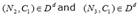are transferred from the direct shipment delivery system to the hub-and-spoke delivery system, such as N2 è N1è C1 and N3 è N1è C1, the total rescue cost will be reduced 10.75% when compared with the cost before adjustment (4090/3650). Similarly, we can complete the whole operations according to the solution procedure. Replenishment arcs that need to be transferred in each cycle are shown in Table 4. Total rescue cost at each cycle is presented in Figure 5.

 Cycle Arcs need to be transferred Cycle Arcs need to be transferred Before After Before After t=0 N2gC1 N3gC1 N2gN1gC1 N3g N1gC1 t=1 N2gC1 N3gC1 N2gN1gC1 N3gN1gC1 t=2 N2gC1 N2gN1gC1 t=3 N2gC1 N2gN1gC1 *There is no adjustment when t=4,5,6,7,8,9,10

Table 4. Transferred arcs in each rescue cycle

Figure 5 shows the change in total rescue cost over time. From this figure, we can get the following two conclusions: (1) Coupled with Figure 4, we can see that demand for medical resources is decreasing, which implies that epidemic diffusion is on the recovered stage. (2) Coupled with Table 4, we can see that the total rescue cost can be reduced in a certain degree by using the proposed heuristic algorithm. It is worth mentioning that there is no adjustment after rescue cycle t=4, for that NSSs and UHDs which are adjacent to the epidemic areas will have stored enough resources at that time.Figure 5. Total rescue cost at each rescue cycle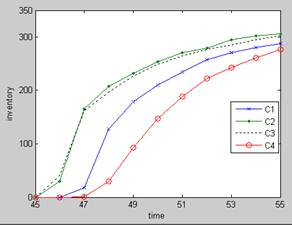Figure 6. Inventory level in different UHD over time

Figure 6 shows the inventory level in different UHDs. It shows that inventory level in each UHD has been improved and raised as time goes by. Therefore, with the application of the three-level and dynamic optimization model, the total rescue cost can be controlled effectively, and meanwhile, inventory level in each UHD can be restored and raised gradually. Thus, such optimization model achieves a win-win rescue effect.

5. Conclusions

In this article, we develop a discrete time-space network model to study the medical resources allocation problem in the recovered stage when an epidemic outbreak. In each decision-making cycle, the allocation of medical resources across the region from NSSs through UHDs and ADPCs to EDHs is determined by a linear programming model with the dynamic demand that is forecasted by an epidemic diffusion rule. The novelty of our model against the existing works in literature is characterized by the following three aspects:

(1) While most research on medical resources allocation studies a static problem taking no consideration of the time evolution and dynamic nature of the demand, the model proposed in this paper addresses a time-series demand that is forecasted in match of the course of an epidemic diffusion. The model couples a multi-stage linear programming for optimal allocation of medical resources with a proactive forecasting mechanism cultivated from the epidemic diffusion dynamics. The rationale that the medical resources allocated in early periods will take effect in subduing the spread of the epidemic spread and thus impact the demand in later periods has been for the first time incorporated into our model.

(2) A win-win emergency rescue effect is achieved by the integrated and dynamic optimization model. The total rescue cost is controlled effectively, and meanwhile, inventory level in each UHD is restored and raised gradually.

(3) In this article, the medicine logistics operation problem has been decomposed into several mutually correlated sub-problems, and then be solved systematically in the same decision scheme. Thus, the result will be much more suitable for real operations.

As the limitation of the model, it is developed for the medical resources allocation in a geographic area where an epidemic disease has been spreading and it does not consider possible cross area diffusion between two or more geographic areas. We assume that once an epidemic outbreak, the government has effective means to separate the epidemic areas so that cross-area spread can be basically prevented. However, this cannot always be guaranteed in reality.

Acknowledgments

This work has been partially supported by the MOE (Ministry of Education in China) Project of Humanities and Social Sciences (No. 11YJCZH109), and by the Fundamental Research Funds for the Central UniversitiesNo. NUST (2011YBXM96), (2011XQTR10), (AE88072) and (JGQN1102). The authors wish to thank Prof. Ding Zhang from State University of New York (USA), who read earlier versions of this paper. His helpful suggestions improved the presentation significantly.

References

Craft, D.L., Wein, L.M., & Alexander, H.W. (2005). Analyzing bioterror response logistics: the case of anthrax. Management Science, 51, 679-694. http://dx.doi.org/10.1287/mnsc.1040.0348

Han, X.P. (2007). Disease spreading with epidemic alert on small-world networks. Physics Letters A, 365, 1-5. http://dx.doi.org/10.1016/j.physleta.2006.12.054

Jung, E., Iwami, S. & Takeuchi, Y. (2009). Optimal control strategy for prevention of avian influenza pandemic. Journal of Theoretical Biology, 260, 220-229. http://dx.doi.org/10.1016/j.jtbi.2009.05.031

Kaplan, E.H., Craft, D.L., & Wein, L.M. (2002). Emergency response to a smallpox attack: the case for mass vaccination. Proceedings of the National Academy of Sciences, 99, 10935-10940. http://dx.doi.org/10.1073/pnas.162282799

Liu J.Y., Li C.L., & Chan C.Y. (2003). Mixed truck delivery systems with both hub-and-spoke and direct shipment. Transportation Research Part E, 39, 325-339. http://dx.doi.org/10.1016/S1366-5545(03)00005-X

Liu, M., & Zhao, L.D. (2009). Optimization of the emergency materials distribution network with time windows in anti-bioterrorism system. International Journal of Innovative Computing, Information and Control, 5, 3615-3624. http://www.ijicic.org/isii08-064-1.pdf

Liu, M., & Zhao, L.D. (2011). Analysis for epidemic diffusion and emergency demand in an anti-bioterrorism system. International Journal of Mathematical Modelling and Numerical Optimisation, 2, 51-68. http://dx.doi.org/10.1504/IJMMNO.2011.037199

Liu, M., & Zhao, L. D. (2012). An integrated and dynamic optimisation model for the multi-level emergency logistics network in anti-bioterrorism system. International Journal of Systems Science, 43, 1464-1478. http://dx.doi.org/10.1080/00207721.2010.547629

Qiang, P., & Nagurney, A. (2012). A bi-criteria indicator to assess supply chain network performance for critical needs under capacity and demand disruptions. Transportation Research Part A: Policy and Practice, 46(5), 801-812. http://dx.doi.org/10.1016/j.tra.2012.02.006

Rachaniotis, N.P., Dasaklis, T.K., & Pappis, C.P. (2012). A deterministic resource scheduling model in epidemic control: A case study. European Journal of Operational Research, 216, 225-231. http://dx.doi.org/10.1016/j.ejor.2011.07.009

Saramäki, J., & Kaski, K. (2005). Modeling development of epidemics with dynamic small-world networks. Journal of Theoretical Biology, 234, 413-421. http://dx.doi.org/10.1016/j.jtbi.2004.12.003

Sheu, J.B. (2007). An emergency logistics distribution approach for quick response to urgent relief demand in disasters. Transportation Research Part E, 43, 687-709. http://dx.doi.org/10.1016/j.tre.2006.04.004

Wang, H.Y., Wang, X.P., & Zeng, A.Z. (2009). Optimal material distribution decisions based on epidemic diffusion rule and stochastic latent period for emergency rescue. International Journal of Mathematics in Operational Research, 1, 76-96. http://dx.doi.org/10.1504/IJMOR.2009.022876

Wein, L.M., Liu, Y.F., & Leighton, T.J. (2005). Evaluation of a HEPA/vaccine plan for indoor remediation after an airborne anthrax attack. Emerging Infectious Diseases, 11, 69-76. http://dx.doi.org/10.3201/eid1101.040635

Xu, X.J., Peng, H.O., Wang, X.M., & Wang, Y.H. (2006). Epidemic spreading with time delay in complex networks. Physica A, 367, 525-530. http://dx.doi.org/10.1016/j.physa.2005.11.035

Yan, S.Y., & Shih, Y.L (2009). Optimal scheduling of emergency roadway repair and subsequent relief distribution. Computers & Operations Research, 36, 2049-2065. http://dx.doi.org/10.1016/j.cor.2008.07.002

Zhu, Y.F., Gu, L. Yu, J.X. et al. (2009). Analysis on the Epidemiological Characteristics of Escherichia coli O157:H7 Infection in Xuzhou, Jiangsu, China, 1999. Journal of Nanjing Medical University (English Edition), 23, 20-24. http://dx.doi.org/10.1016/S1007-4376(09)60021-6